# Python大数据-对淘宝用户的行为数据分析

1、读取查看数据的基本信息和数据的完整性

2、一致化处理：

3、查看是否有缺失值

1、不同时间下PV、UV的流量变化情况

2、不同购物行为在不同时间维度下的变化情况

六、结论分析

## 一、项目背景

• 通过对2014年11月17日至2014年12月17日之间数据进行合并，获得该月用户的行为数据，数据量在一千万条左右。
• 本次数据是在网上获取的来源于：数据集-阿里云天池，不在进行抓取或收集，大家可以看这篇文章Python爬虫-抓取数据到可视化全流程的实现，详细的写了数据抓取的过程

• 使用的主要工具：python --jupyter notebook

## 二、项目目标

• 用户行为分析是对用户在产品上的产生的行为及行为背后的数据进行分析，通过本次分析希望可以实现：

• 1、通过构建用户行为模型和用户画像，来改变产品决策，实现精细化运营，指导业务增长

2、 在产品运营过程中，对用户行为的数据进行收集、存储、跟踪、分析与应用等，可以找到实现用户自增长的存在的问题、群体特征与目标用户

# 三、分析思路

• 主要从以下四个维度对用户行为进行分析和建议：
• 1、用户的行为习惯分析：利用pv、uv等指标，分析用户活跃的时间段和趣事，熟悉用户行为的时间模式；
• 2、用户的行为转换情况分析：通过采用漏斗模型从单击浏览到支付购买的各个阶段对用户行为进行分析，确定各个环节的流失率，并提出相应的改善建议；
• 3、用户偏好分析：根据商品的点击、收藏、加购、购买频率，探索用户对商品的购买偏好，制定对不同商品、不同用户之间的营销策略；
• 4、核心用户分析：找出最具有价值的核心用户群，考虑针对该群体的营销策略，针对这个群体用户行为推送个性化推送，优惠券等。

## 四、数据清洗

• 首先该数据是在多个文件下的CSV文件，通过递归对文件夹内所有文件进行查询，并合并了所有的指定文件，具体的操作步骤大家可以看Python遍历目录下的所有文件、读取、千万条数据合并详解这篇文章，里边详细的写了如何遍历所有文件夹，如何组合，如何批量的打开文件目录下的所有文件，并对多文件下的文件进行合并，本文就不再赘述数据合并的过程了，直接采用合并后的数据，大家不懂可以看我的以上两盘博客，写的很详细。
• ### 1、读取查看数据的基本信息和数据的完整性

• data=final_data
data.head()

查看一下我们合并后表格的情况，可以发现目前表格的列数为7列

•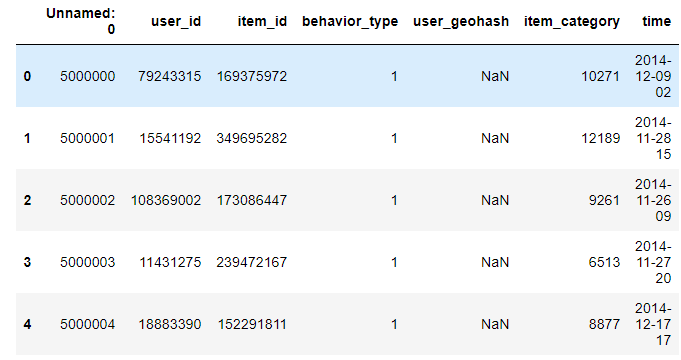​

其中'Unnamed: 0','user_geohash'（有缺失）两列数据我们在分析时不涉及，对这两列数据进行删除

• import pandas as pd
final_data.drop(['Unnamed: 0','user_geohash'],axis=1,inplace=True)
final_data.head()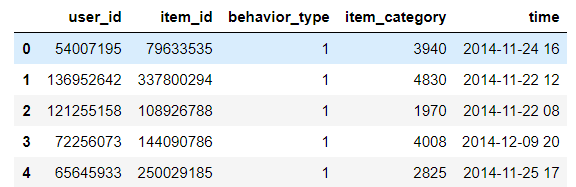​

成功进行删除，检查数据类型

• data.dtypes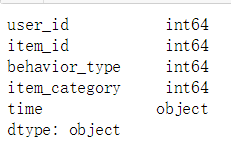### 2、一致化处理：

• 可以发现目前time是object型的，因为分析涉及到时间、天、小时，所以，要把数据集里的时间戳列，即time_stamp列转化为日期。。

• data['date'] = data['time'].map(lambda x:x.split(' '))
data['hour'] = data['time'].map(lambda x:x.split(' '))
data['date']=pd.to_datetime(data['date'])
data['hour'] = data['hour'].astype('int32')
data.dtypes
•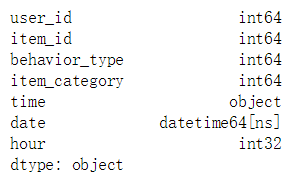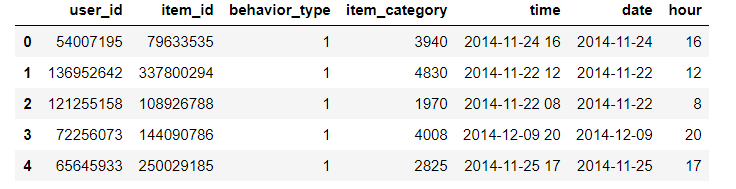可以看到目前date和Hour的类型已经成功转换，符合我们的预期，查看表格中是否有空数据，并查看一下表格的量
• ### 3、查看是否有缺失值

• data.isnull().sum()
data.shape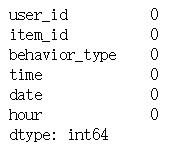​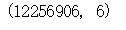​

可以看到数据中并没有空数据，数据的规模在1000万左右，分为6列，依次为用户id、商品id、用户行为类型、时间。其中用户行为类型中1代表点击（当做pv），2代表collect（收藏），3代表cart（加入购物车）数据较为完整，不需要继续进行清洗，对数据进行分析

## 五、数据分析

• 流量指标分析：流量指标是指用户在某一个网站操作的每一个步骤记录的指标，埋点数据，PV是指其浏览量，UV代表独立访客数，访问深度代表每个独立访客的浏览量，页面跳出率 则是指浏览某个页面离开的次数/这个页面的全部访问次数

## 1、不同时间下PV、UV的流量变化情况

1）每天的PV、UV变化情况

• 首先计算一下总流量
• # 总PV值=数据条数
import pandas as pd
import numpy as np
import matplotlib.pyplot as plt
import os
data.shape
总流量为12256906，在计算一下日平均流量、日平均独立访客数
• ##日PV
pv_daily = data.groupby(['date'])['user_id'].count().reset_index().rename(columns={'user_id':'pv_daily'})
pv_daily.head()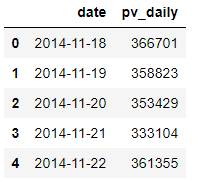• 日平均独立访客数与日平均流量的区别在于要进行去重

##日UV
uv_daily = data.groupby(['date'])['user_id'].apply(lambda x:x.drop_duplicates().count()).reset_index().rename(columns={'user_id':'uv_daily'})
s=uv_daily['uv_daily']
pv_daily['uv_daily']=s
pv_daily

将两表合并

•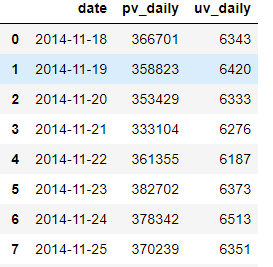• plt.figure(figsize=(40,20),dpi=80)

font={
"family":"kaiti",
"size":'30'
}
plt.rc("font",**font)
plt.subplot(211)#在第一个位置日平均流量图
plt.plot(pv_daily['date'],pv_daily['pv_daily'],'co-')
plt.gca().xaxis.set_major_formatter(mdates.DateFormatter('%m/%d'))
plt.gca().xaxis.set_major_locator(mdates.DayLocator())    # 按月显示,按日显示的话，将MonthLocator()改成DayLocator()
plt.gcf().autofmt_xdate()
ax=plt.gca()
ax.spines["top"].set_color("w")
ax.spines["bottom"].set_color("r")
ax.spines["left"].set_color("r")
ax.spines["right"].set_color("w")
plt.gcf().autofmt_xdate()
#设置X轴标签
plt.xlabel("时间")
#设置y轴标签
plt.ylabel("日平均流量统计图")
plt.title('日平均流量')
plt.figure(figsize=(40,20), dpi=80)
plt.subplot(212)#第二个位置绘制日平均独立访客数
plt.plot(pv_daily['date'],pv_daily['uv_daily'],'yo-')
plt.gca().xaxis.set_major_formatter(mdates.DateFormatter('%m/%d'))
plt.gca().xaxis.set_major_locator(mdates.DayLocator())
ax=plt.gca()
ax.spines["top"].set_color("w")
ax.spines["bottom"].set_color("r")
ax.spines["left"].set_color("r")
ax.spines["right"].set_color("w")
plt.title('日独立访问客流量')
plt.gcf().autofmt_xdate()
#设置X轴标签
plt.xlabel("时间")
#设置y轴标签
plt.ylabel("日独立访客量统计图")
plt.show()

绘制子图，将日平均流量和独立访问客数放在一起进行对比分析：

•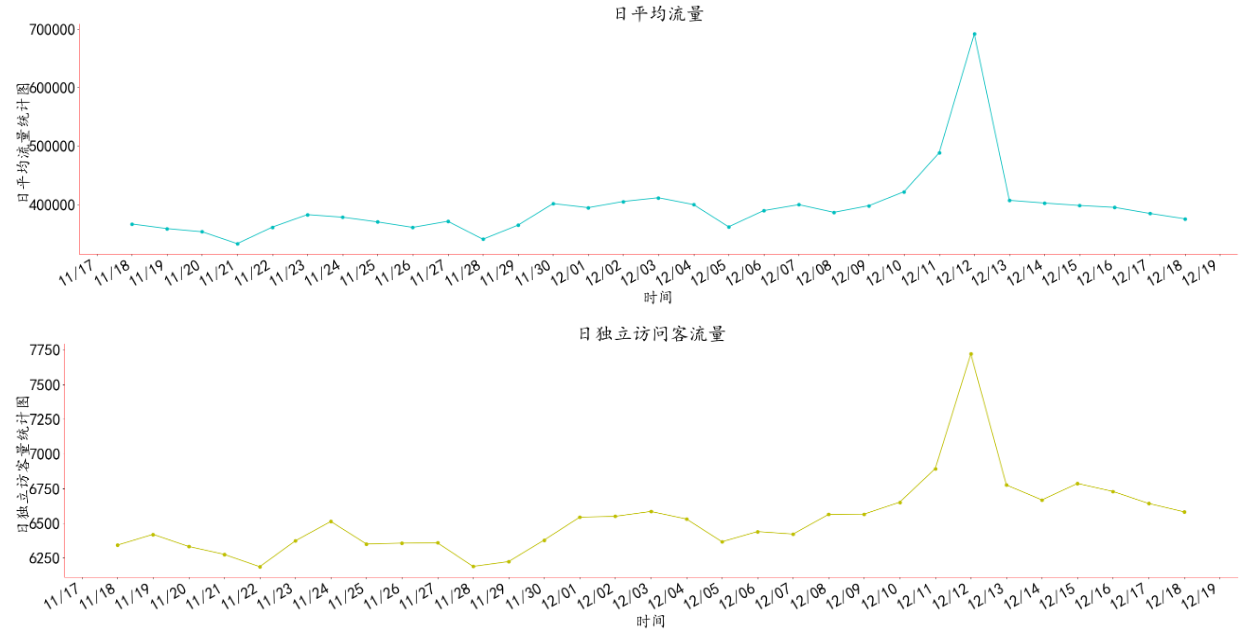• 可以发现在双十二当天是流量和独立访客数的高峰，在平常波动不大

# 每天的时刻数据
pv_daily_hour = data.groupby(['hour'])['user_id'].count().reset_index().rename(columns={'user_id':'pv'})
uv_daily_hour = data.groupby(['hour'])['user_id'].apply(lambda x:x.drop_duplicates().count()).reset_index().rename(columns={'user_id':'uv'})
uv_daily_hour.head()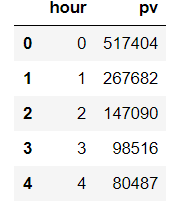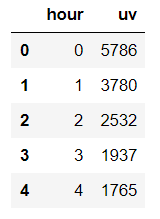plt.figure(figsize=(15,18),dpi=80)
plt.subplot(211)
plt.plot(pv_daily_hour['hour'],pv_daily_hour['pv'],'bo-')
plt.title("每小时PV")
plt.savefig("每小时PV.png")
plt.xticks(np.arange(0, 24, step=1))
plt.xlim(data.index.values)
plt.figure(figsize=(15,18),dpi=80)
plt.subplot(212)
plt.plot(uv_daily_hour['hour'],uv_daily_hour['uv'],'yo-')
plt.title("每小时UV")
plt.savefig("每小时UV.png")
plt.xticks(np.arange(0, 24, step=1))
plt.xlim(data.index.values)
plt.show()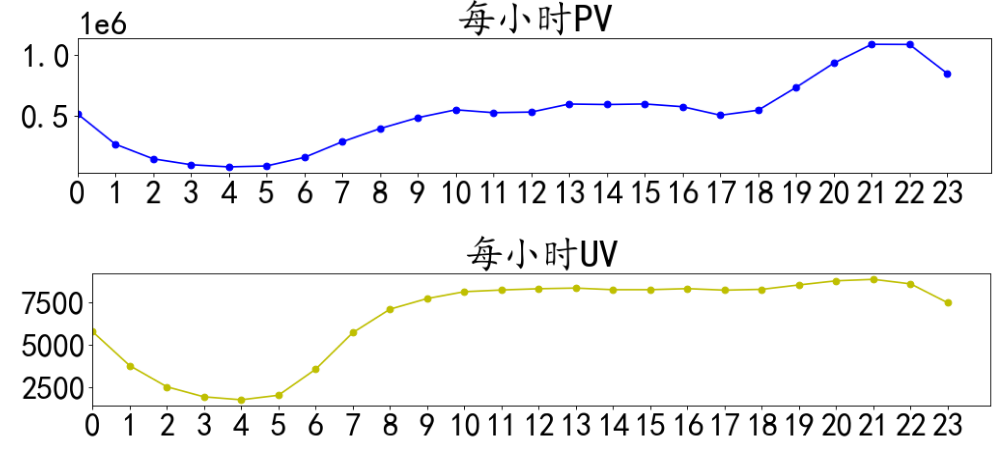•  从早上5：00-10:00,18:00-21:00这两个时间段pv有较明显上升；uv从早上6:00-10:00有较明显增加，而后到21点uv保持稳定数量，然后开始下降；pv、uv变化符合大众工作作息时间，侧面证明数据是真是有效的。

## 2、不同购物行为在不同时间维度下的变化情况

plt.figure(figsize=(10, 4))
sns.lineplot(data=d_pv_h, lw=3)
plt.show()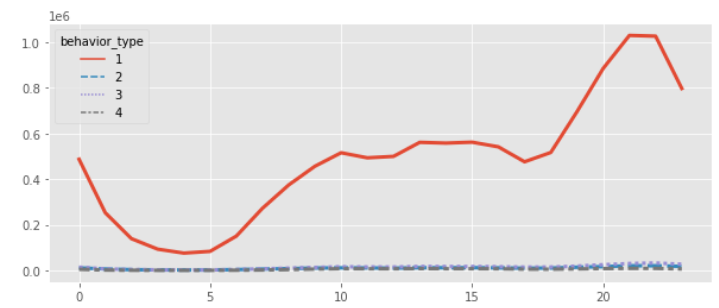plt.figure(figsize=(10, 4))
sns.lineplot(data=d_pv_h.iloc[:, 1:], lw=3)
plt.show()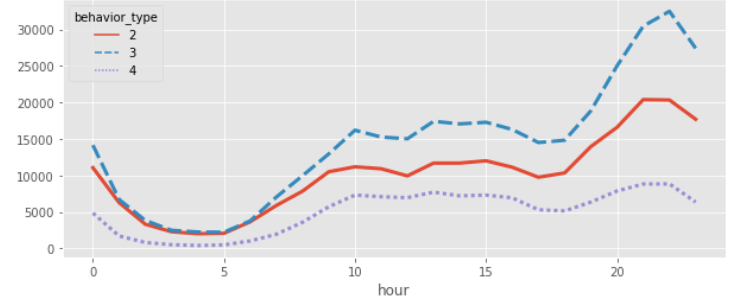虽然大体上各波动趋势相同，但是加购物车数远高于收藏数。

## 每个UV的平均访问深度=总流量/独立访客数
round(data['user_id'].shape/data['user_id'].nunique(),2)
##=1225.69
## 每个UV的日平均访问深度
round(data['user_id'].shape/data['user_id'].nunique()/data['date'].nunique(),2)
##=39.54

## 3 、用户转化行为漏斗模型分析

## 计算每一个行为环节用户的访问量
view = data.groupby(['behavior_type'])['user_id'].count().reset_index().rename(columns={'user_id':'pv'})
view.head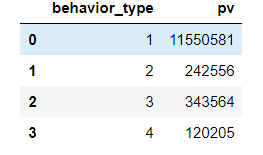beihavior_type - 1 点击 2 收藏 3 加购物车 4 支付

#计算各个环节的流失率
print("点击->加购物车流失率是:%d" % round((view['pv']-view['pv'])*100/view['pv'],4) + '%')
print('点击->收藏流失率是:%d'  % round((view['pv']-view['pv'])*100/view['pv'],4) + '%')
print('加购物车->支付的流失率是:%d' % round((view['pv']-view['pv'])*100/view['pv'],4) + '%')
print('收藏->支付的流失率是:%d' % round((view['pv']-view['pv'])*100/view['pv'],4) + '%')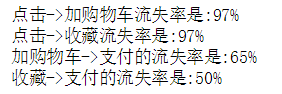from pyecharts.charts import Funnel
attr = ['点击','收藏','加购物车','支付']
# 数据支持[(属性,数量)]
image_data = [(attr[i],int(view['pv'][i])) for i in range(len(attr))]
print(image_data)

funnel.render_notebook()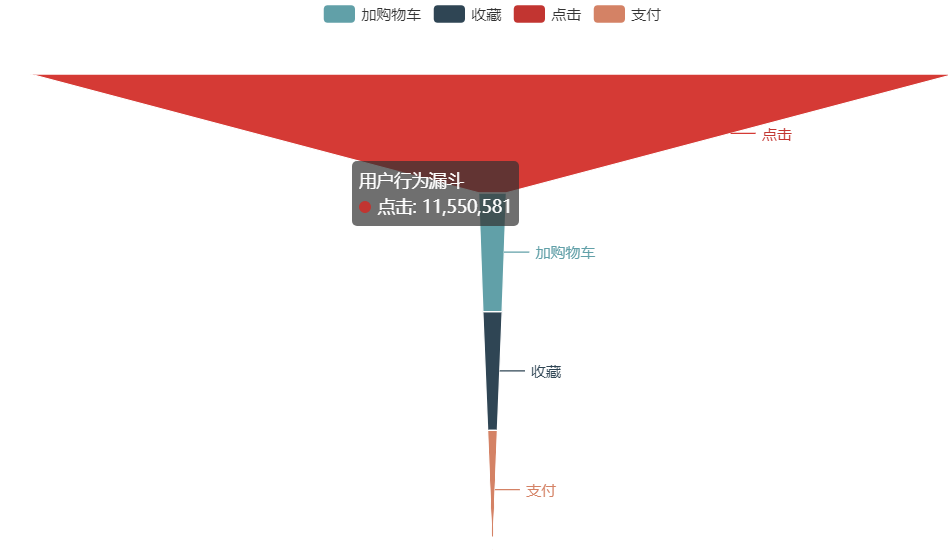用户产生点击后可能进行的操作分别为：点击->加购物车、点击->收藏、加购物车->支付、收藏->支付，可以明显的看出用户的流失率比较大，根据用户购买途径计算出各个阶段用户流失率：• 从浏览——加入购物车/收藏——付款的转化率较低；可以看出浏览到加入购物车或者收藏这一环节的流失率较大，可能由于产品不符合消费者需求或者详情页面不友好等需要对其中原因进一步挖掘分析，查看独立访客情况。

view = data.groupby(['behavior_type'])['user_id'].apply(lambda x:x.drop_duplicates().count()).reset_index().rename(columns={'user_id':'pv'})
view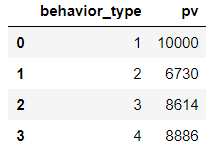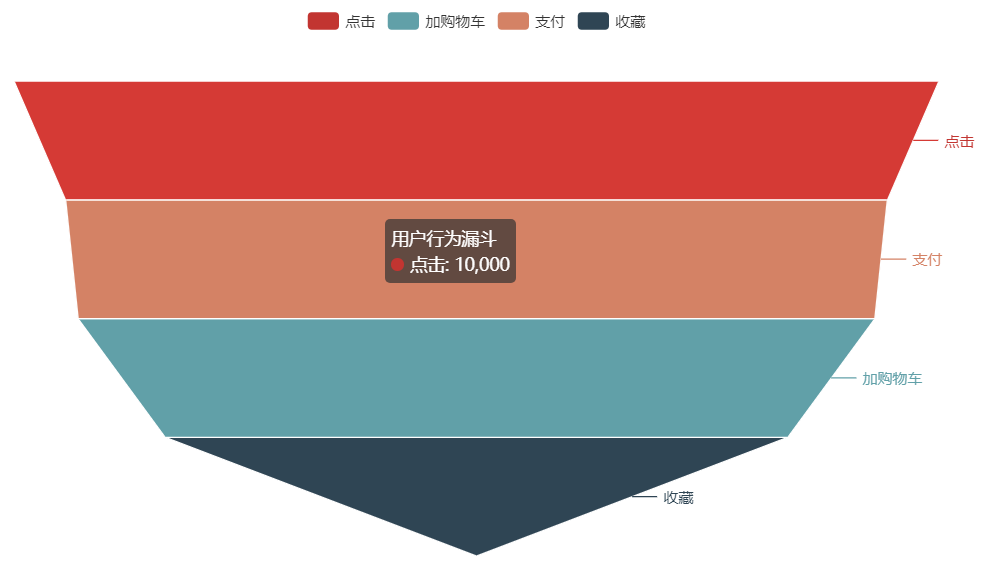##计算每天的购买数量
df = data[data['date']!='2014-12-12']
##计算每小时的购买数量
hour_buy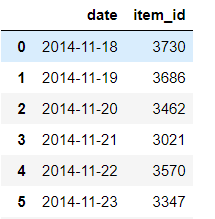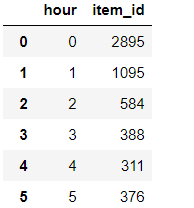plt.figure(figsize=(20,5))
plt.xticks(rotation=30)
plt.title('按日期观察成交量')
plt.savefig("按日期观察成交量.png")
plt.show()plt.figure(figsize=(20,5))
plt.xticks(rotation=30)
plt.title('按时段观察成交量')
plt.savefig("按时段观察成交量.png")
plt.show()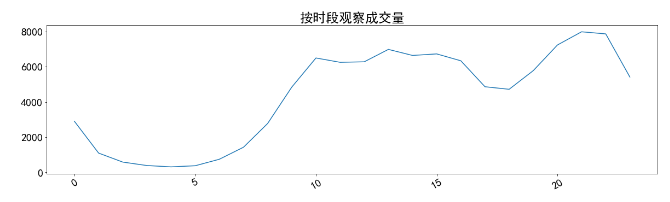用户转化行为漏斗模型分析

## 六、结论分析

• 本次数据中一个月内的访问用户总数为（UV)：29233，页面总访问量为（PV）：2685348，平均每人每周访问量为91.8次页面
• 从浏览——加入购物车/收藏——付款的转化率较低；可以看出浏览到加入购物车或者收藏这一环节的流失率较大，可能由于产品不符合消费者需求或者详情页面不友好等需要对其中原因进一步挖掘分析；
•  从早上5：00-10:00,18:00-21:00这两个时间段pv有较明显上升；uv从早上6:00-10:00有较明显增加，而后到21点uv保持稳定数量，然后开始下降；pv、uv变化符合大众工作作息时间，侧面证明数据是真是有效的；
• 双十二期间pv、uv远高于平时，且付款人数高出收藏人数许多
• 后续可通过聚类分析来对用户群体进行分类，从而实施更精确的营销策略；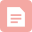博客

03-271333博客

08-02263博客
python分析1225万条淘宝数据，终于搞清楚了我交易行为！超长
05-15305博客

02-22191博客
Python 爬虫实战之爬淘宝商品并做数据分析
09-172100博客

03-261016博客

03-203319博客

07-164337博客

04-132858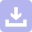下载

07-28博客
python爬取商品信息_python爬取并分析淘宝商品信息
11-21800博客
Python分析用户消费行为
06-177737博客

06-221198博客

10-01807博客

10-12355博客

04-226248博客

05-242836博客
python基于淘宝历史数据用户行为分析
03-258033博客

08-027471

### “相关推荐”对你有帮助么？

•非常没帮助
•没帮助
•一般
•有帮助
•非常有帮助

©️2022 CSDN 皮肤主题：Age of Ai 设计师：meimeiellie¥2 ¥4 ¥6 ¥10 ¥20余额支付 (余额：-- )扫码支付获取中扫码支付点击重新获取扫码支付1.余额是钱包充值的虚拟货币，按照1:1的比例进行支付金额的抵扣。
2.余额无法直接购买下载，可以购买VIP、C币套餐、付费专栏及课程。余额充值